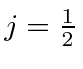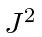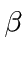## Adding Spinto Integer Orbital Angular Momentum

Our goal is to add orbital angular momentum with quantum numberto spin. We can show in several ways that, for, that the total angular momentum quantum number has two possible valuesor.For, onlyis allowed. First lets argue that this makes sense when we are adding two vectors. For example if we add a vector of length 3 to a vector of length 0.5, the resulting vector could take on a length between 2.5 and 3.5 For quantized angular momentum, we will only have the half integers allowed, rather than a continuous range. Also we know that the quantum numbers likeare not exactly the length of the vector but are close. So these two values make sense physically.

We can also count states for each eigenvalue ofas in the following examples.

* Example: Counting states forplus spin.*
* Example: Counting states for anyplus spin.*
As in the last section, we could start with the higheststate,, and apply the lowering operator to find the rest of the multiplet with. This works well for some specificbut is hard to generalize.

We can work the problem in general. We know that each eigenstate ofandwill be a linear combination of the two product states with the right.The coefficientsandmust be determined by operating with.We have made a choice in how to write these equations:must be the same throughout. The negativestates are symmetric with the positive ones. These equations will be applied when we calculate the fine structure of Hydrogen and when we study the anomalous Zeeman effect.

Jim Branson 2013-04-22